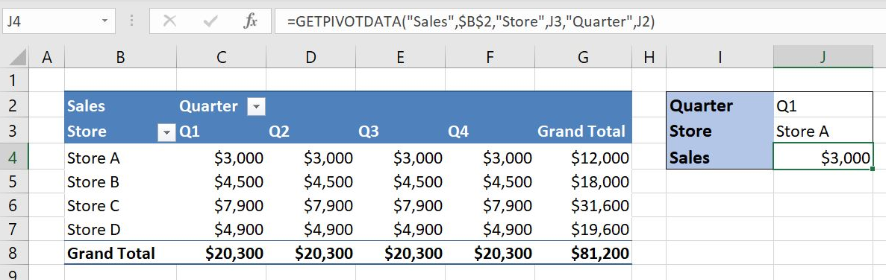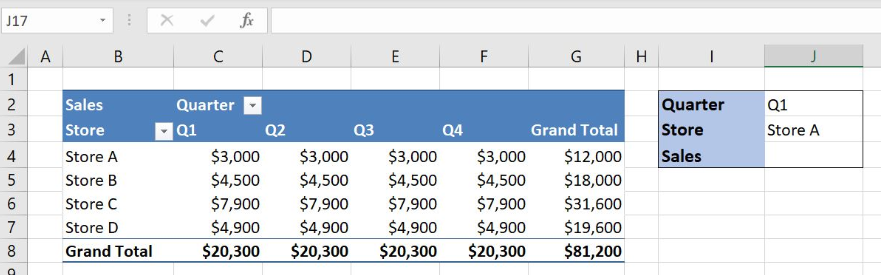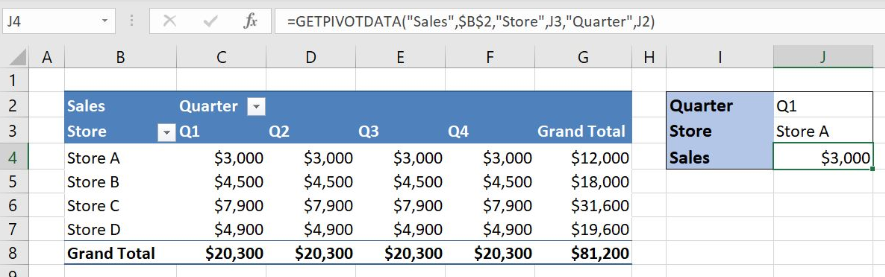Get instant live expert help with Excel or Google Sheets“My Excelchat expert helped me in less than 20 minutes, saving me what would have been 5 hours of work!”

#### Post your problem and you’ll get expert help in seconds.

Your message must be at least 40 characters
Our professional experts are available now. Your privacy is guaranteed.

# Excel GETPIVOTDATA Function

Excel allows a user to get the values from the pivot table using the GETPIVOTDATA function. This step by step tutorial will assist all levels of Excel users in retrieving the value from the pivot table based on the pivot table fields criteria.Figure 1. The result of the GETPIVOTDATA function

## Syntax of the GETPIVOTDATA Formula

The generic formula for the GETPIVOTDATA function is:

`=GETPIVOTDATA(data_field, pivot_table, [field1,item1], ...)`

The parameters of the GETPIVOTDATA function are:

• data_field – a name of the pivot table value field from which we want to get the data
• pivot_table – a reference to a cell or a cell range in a pivot table
• field1 – a field to refer to
• Item1 – an item of the field to refer to

## Setting up Our Data for the LOOKUP Function

The first table is a pivot table and consists of: “Quarter” (Column field in the pivot table), “Store” (Row field in the pivot table) and “Sales” (Value field in the pivot table). The second table has data for “Quarter” and “Store” that exist in the pivot table and an empty space for the “Sales” value. Based on the “Quarter” and “Store” values from the second table we want to retrieve “Sales” value from the pivot table and to place the result in the cell J4.Figure 2. The tables for the GETPIVOTDATA function example

## Get a Sales Value for Quarter and Store with GETPIVOTDATA Function

In our example, we want to get a sales value from the pivot table based on the quarter and the store from the second table.

The formula looks like:

`=GETPIVOTDATA("Sales",\$B\$2,"Store",J3,"Quarter",J2)`

The parameter data_field is “Sales”, the name of the pivot table value field while the pivot_table is the cell B2. The field1 and the field2 are the names of the pivot table fields “Store” and “Quarter”. Item1 and Item2 are the cells J3 and J2, field items Q1 and Store A for which we want to get the sales value.

To apply the GETPIVOTDATA function, we need to follow these steps:

• Select cell J4 and click on it
• Insert the formula: `=GETPIVOTDATA("Sales",\$B\$2,"Store",J3,"Quarter",J2)`
• Press enterFigure 3. Using the GETPIVOTDATA function to get a Sales value based on the Quarter and the Store

As we want to get the sales values from the pivot table, the data_field argument will be “Sales” value field from the pivot table. The pivot_table argument can be the reference to any cell in the pivot table.

Field arguments are the names of the pivot table fields “Store” and “Quarter” based on which we want to get the sales data. Item fields are defined in the cells J3 and J2 because we want to get the data for the Store A and the quarter Q1. Formula result is \$3,000, a sales value for the Store A and Q1.

Most of the time, the problem you will need to solve will be more complex than a simple application of a formula or function. If you want to save hours of research and frustration, try our live Excelchat service! Our Excel Experts are available 24/7 to answer any Excel question you may have. We guarantee a connection within 30 seconds and a customized solution within 20 minutes.

### Did this post not answer your question? Get a solution from connecting with the expert.Another blog reader asked this question today on Excelchat:
Solution examplesI'm trying to figure out how to have a value found in column c placed into column d if the value in column a equals that found in column b. Does that make sense?
Solved by I. H. in 40 minsWhen i do a VLOOKUP, I cannot autofill the formula for the rest of the table. It copies the initial cell that the VLOOKUP was done.
Solved by G. A. in 20 minsI need a formula (I think VLOOKUP) that will enable me to: Use data from one column, compare with another and tell me the difference. Im a teacher and want to work out the amount of marks needed to the next grade boundary. I've created the rest, but this has me stumped!
Solved by F. A. in 28 minshow do I do the same formula for different cells, but i want a different cells value in each of the formulas. For example, if i was trying to find the percentage grade of each student in a class of 300.
Solved by I. W. in 14 minsI'm not sure as to why my formula isn't working. I will send two files... Workbook 1.xlsx.xls Workbook 2.xlsm The problem is in Column H of Workbook 1.
Solved by E. H. in 42 mins## Subscribe to Excelchat.coAnother blog reader asked this question today on Excelchat: